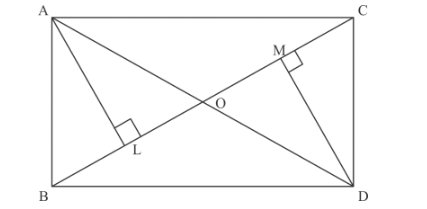# In Fig. 8, and ΔDBC are on the same base BC and on`
Question:

In Fig. 8, and ΔDBC are on the same base BC and on opposite sides of BC ad Q is the point of intersection of AD and BC.

Prove that $\frac{\operatorname{area}(\Delta A B C)}{\operatorname{area}(\Delta D B C)}=\frac{A O}{D O}$

Solution:

We have given the diagram in which

We have to prove that $\frac{\text { Area of } \triangle A B C}{\text { Area of } \triangle D B C}=\frac{A O}{D O}$

Firstly, we draw a line from A perpendicular to line BC and after that we draw a line from D perpendicular to BC.From the above figure we can easily see that theandare similar

Therefore by the properties of similar triangle, we have

$\frac{A L}{D M}=\frac{A O}{D O} \ldots \ldots$(1)

Now,

$\frac{\text { Area of } \triangle A B C}{\text { Area of } \triangle D B C}=\frac{\frac{1}{2} B C \cdot A L}{\frac{1}{2} B C \cdot D M}$

$=\frac{A L}{D M}$

From equation (1), we get

$\frac{\text { Area of } \triangle A B C}{\text { Area of } \triangle D B C}=\frac{A O}{D O}$

Hence proved.